Courses

Test: Discrete Time Signal Analysis - 1

15 Questions MCQ Test Digital Signal Processing | Test: Discrete Time Signal Analysis - 1

Description
Attempt Test: Discrete Time Signal Analysis - 1 | 15 questions in 15 minutes | Mock test for Electrical Engineering (EE) preparation | Free important questions MCQ to study Digital Signal Processing for Electrical Engineering (EE) Exam | Download free PDF with solutions
QUESTION: 1

What is the Fourier series representation of a signal x(n) whose period is N?

Solution:

Explanation: Here, the frequency F0 of a continuous time signal is divided into 2π/N intervals.
So, the Fourier series representation of a discrete time signal with period N is given as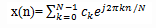where ck is the Fourier series coefficient

QUESTION: 2

What is the expression for Fourier series coefficient ck in terms of the discrete signal x(n)?

Solution:

Explanation: We know that, the Fourier series representation of a discrete signal x(n) is given as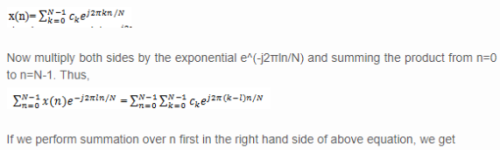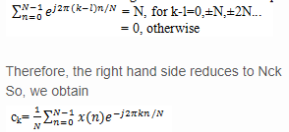QUESTION: 3

Which of the following represents the phase associated with the frequency component of discrete-time Fourier series(DTFS)?

Solution:

Explanation: We know that,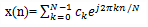In the above equation, ck represents the amplitude and ej2πkn/N represents the phase associated with the frequency component of DTFS.

QUESTION: 4

The Fourier series for the signal x(n)=cos√2πn exists.

Solution:

Explanation: For ω0=√2π, we have f0=1/√2. Since f0 is not a rational number, the signal is not periodic. Consequently, this signal cannot be expanded in a Fourier series.

QUESTION: 5

What are the Fourier series coefficients for the signal x(n)=cosπn/3?

Solution:

Explanation: In this case, f0=1/6 and hence x(n) is periodic with fundamental period N=6.
Given signal is x(n)= cosπn/3=cos2πn/6=1/2 e^(j2πn/6)+1/2 e^(-j2πn/6)
We know that -2π/6=2π-2π/6=10π/6=5(2π/6)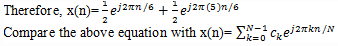So, we get c1=c2=c3=c4=0 and c1=c5=1/2.

QUESTION: 6

What is the Fourier series representation of a signal x(n) whose period is N?

Solution:

Explanation: Here, the frequency F0 of a continuous time signal is divided into 2π/N intervals.
So, the Fourier series representation of a discrete time signal with period N is given aswhere ck is the Fourier series coefficient

QUESTION: 7

What is the average power of the discrete time periodic signal x(n) with period N ?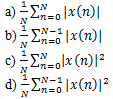Solution:

Explanation: Let us consider a discrete time periodic signal x(n) with period N.
The average power of that signal is given asQUESTION: 8

What is the equation for average power of discrete time periodic signal x(n) with period N in terms of Fourier series coefficient ck?

Solution:

Explanation: We know that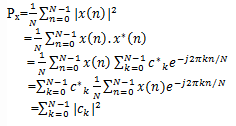QUESTION: 9

What is the Fourier transform X(ω) of a finite energy discrete time signal x(n)?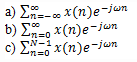d) None of the mentioned

Solution:

Explanation: If we consider a signal x(n) which is discrete in nature and has finite energy, then the Fourier transform of that signal is given as

QUESTION: 10

What is the period of the Fourier transform X(ω) of the signal x(n)?

Solution:

Explanation: Let X(ω) be the Fourier transform of a discrete time signal x(n) which is given as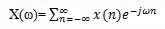Now
So, the Fourier transform of a discrete time finite energy signal is periodic with period 2π.

QUESTION: 11

What is the synthesis equation of the discrete time signal x(n), whose Fourier transform is X(ω)?

Solution:

Explanation: We know that the Fourier transform of the discrete time signal x(n) is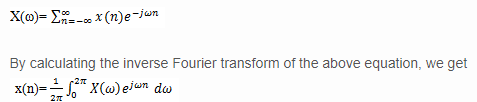The above equation is known as synthesis equation or inverse transform equation.

QUESTION: 12

What is the value of discrete time signal x(n) at n=0 whose Fourier transform is represented as below?

Solution:

Explanation: We know that,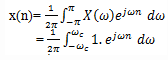At n = 0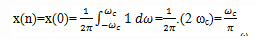Therefore, the value of the signal x(n) at n=0 is ω_c/π.

QUESTION: 13

What is the value of discrete time signal x(n) at n≠0 whose Fourier transform is represented as below?

Solution:

Explanation: We know that,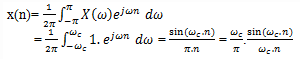QUESTION: 14

The oscillatory behavior of the approximation of XN(ω) to the function X(ω) at a point of discontinuity of X(ω) is known as Gibbs phenomenon.

Solution:

Explanation: We note that there is a significant oscillatory overshoot at ω=ωc, independent of the value of N. As N increases, the oscillations become more rapid, but the size of the ripple remains the same. One can show that as N→∞, the oscillations converge to the point of the discontinuity at ω=ωc. The oscillatory behavior of the approximation of XN(ω) to the function X(ω) at a point of discontinuity of X(ω) is known as Gibbs phenomenon.

QUESTION: 15

What is the energy of a discrete time signal in terms of X(ω)?

Solution:

Explanation: We know that,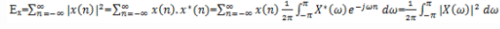Use Code STAYHOME200 and get INR 200 additional OFF Use Coupon Code

Track your progress, build streaks, highlight & save important lessons and more!

Similar ContentRelated tests